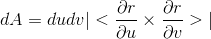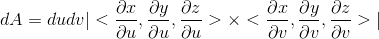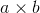# Surface element -- why parallelograms?

• DivergentSpectrum
The cross product is just the length of one of those sides times the length of the other side times the sine of the angle between them. Here, the length of both sides is the same, \sqrt{1+ b^2}, and the angle between them is NOT 90 degrees but the angle between the two vectors, which is the same as the angle between the two lines, which is the same as the angle between the two planes, which is the same as the angle between the two normal vectors. The length of the cross product is the area of the parallelogram.In summary, the surface element of x(u,v), y(u,v), z(u,v) is the absolute value of the cross product between the

#### DivergentSpectrum

we say the surface element of x(u,v),y(u,v),z(u,v) is absolute value of the cross productori don't understand why this is. i know that the absolute value of the cross productis the area of a parallelogram formed by a,b,a+b, but i get lost after that
im guessing something happens where dudv cancels out with ∂u∂v then just leaves ∂r, but i can't really figure what it means.

anyone whos got into computer graphics can tell you triangles are the building blocks of surfaces, because any 3 points occupy the same plane. why a parallelogram then?

Last edited:
Because that is how the maths is simplest.
It makes no difference otherwise since the main difference between above and the computer approximation to a continuous surface is that the parallelogram is arbitrarily small.

Note: the parallelogram is not the only way of viewing the cross product.

DivergentSpectrum said:
i know that the absolute value of the cross productis the area of a parallelogram formed by a,b,a+b, but i get lost after that

We could define a surface integral in a sophisticated way by saying it is the limit of processes that cover the surface with small shapes tangential to its surface and then form a sum of the area of each shape times the value of the function at the point of tangency.

We can define a surface integral in a less sophisticated way by specifying each small shape exactly. For the surface $(x(u,v),y(u,v),z(u,v))$ we can think of $|| <\frac{\partial x}{\partial u}, \frac{\partial y}{\partial u}, \frac{\partial z}{\partial u}> \times <\frac{\partial x}{\partial v}, \frac{\partial y}{\partial v}, \frac{\partial z}{\partial v} >|| du dv$ as

$|| du <\frac{\partial x}{\partial u}, \frac{\partial y}{\partial u}, \frac{\partial z}{\partial u}> \times dv <\frac{\partial x}{\partial v}, \frac{\partial y}{\partial v}, \frac{\partial z}{\partial v} >||$

In other words, think of $du$ and $dv$ as scalars that multiply the vectors involved so the expression is the area of a small parallelogram.

The two gradient vectors conveniently define a plane tangent to the surface. Multiplying the gradient vectors by small scalars when we take the cross product gives us the area of a small parallelogram lying in the tangent plane. This still leaves us open the question of why we can use the gradient vectors without normalizing them each to have length 1. Without that normalization we are using bigger parallelograms where the shape of the surface changes rapidly. It seems we should use smaller parallelograms there instead.

If you start with a rectangle ("$\Delta x$" by "$\Delta y$) in the xy-plane and project onto a plane that is NOT parallel to the xy-plane, the projection is a parallelogram. For example, if the rectangle with vertices at (0, 0), (1, 0), (1, 1), and (0, 1) is projected to the plane z= a+ bx+ cy, the vertices are projected to (0, 0, a), (1, 0, a+ b), (1, 1, a+ b+ c), and (0, 1, a+ c).

It is easy to show that opposite sides of that quadrilateral, such as (0, 0, a) to (1, 0, a+ b) and (0, 1, a+ c) to (1, 1, a+ b+ c) have the same length: $\sqrt{(0- 1)^2+ (0- 0)^2+ (a- (a+ b))^2}= \sqrt{1+ b^2}$ and $\sqrt{(0- 1)^2+ (1- 1)^2+ ((a+ c)- (a+ b+ c)^2}= \sqrt{1+ b^2}$.

But the angles are NOT right angles: the line from (0, 0, a) to (1, 0, a+ b) can be written in parametric equations as x= t, y= 0, z= a+ bt, with t from 0 to 1. A vector in the direction of that line is <1, 0, b>. The line from (0, 0, a) to (0, 1, a+ c) can be written in parametric equations as x= 0, y= t, z= a+ ct with t from 0 to 1. A vector in the direction of that line is <0, 1, c>. The dot product of those two vectors is 0(1)+ 1(0)+ bc= bc, NOT 0.

A quadrilateral with opposite sided of the same length, but angle not right angles, is a general parallelogram.